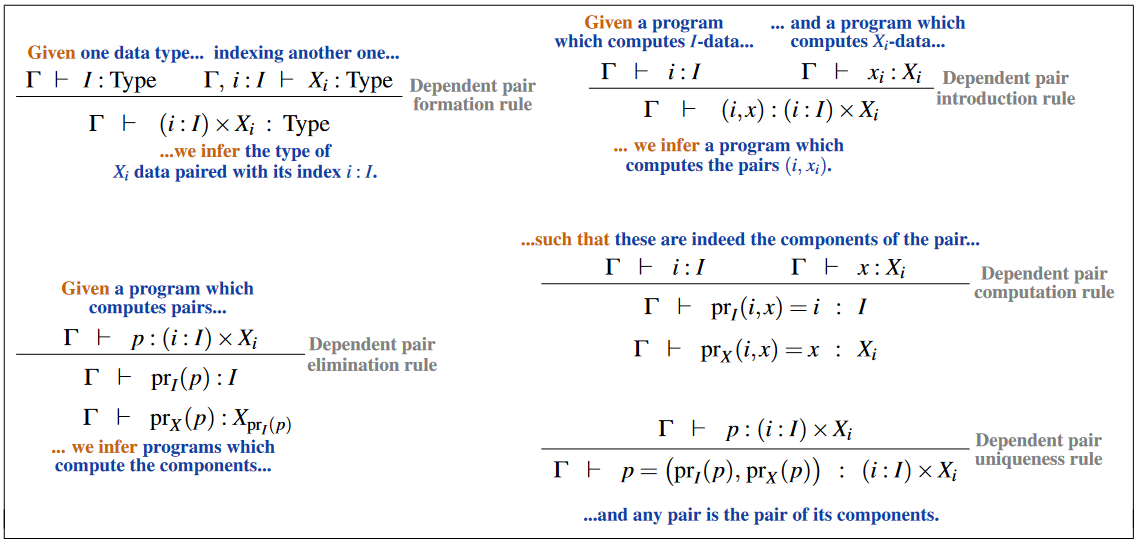# nLab dependent sum

Contents

category theory

## Applications

#### Limits and colimits

limits and colimits

bec

# Contents

## Idea

The dependent sum is a universal construction in category theory. It generalizes the Cartesian product to the situation where one factor may depend on the other. It is the left adjoint to the base change functor between slice categories.

The dual notion is that of dependent product.

## Definition

Let $\mathcal{C}$ be a category and $f \colon A \to I$ a morphism in $\mathcal{C}$ such that pullbacks along $f$ exist in $\mathcal{C}$. These then constitute a base change functor

$f^* \colon \mathcal{C}_{/I} \to \mathcal{C}_{/A}$

between the corresponding slice categories.

###### Definition

The dependent sum or dependent coproduct along the morphism $f$ is the left adjoint $\sum_f \colon \mathcal{C}_{/A} \to \mathcal{C}_{/I}$ of the base change functor

$(\sum_f \dashv f^* ) \colon \mathcal{C}_{/A} \stackrel{\overset{\sum_f}{\to}}{\underset{f^*}{\leftarrow}} \mathcal{C}_{/I} \qquad.$

This is directly seen to be equivalent to the following.

###### Definition

The dependent sum along $f \colon A \to I$ is the functor

$\sum_f \coloneqq f\circ (-) \colon \mathcal{C}_{/A} \to \mathcal{C}_{/I}$

given by composition with $f$.

## Properties

### Relation to the product

Assume that the category $\mathcal{C}$ has a terminal object $* \in \mathcal{C}$. Let $X \in \mathcal{C}$ be any object and assume that the terminal morphism $f \colon X \to *$ admits all pullbacks along it.

Notice that a pullback of some $A \to *$ along $X \to *$ is simply the product $X \times A$, equipped with its projection morphism $X \times A \to X$. We may regard $X \times A \to X$ as the image of the base change functor $f^* \colon \mathcal{C}_{/*} \to \mathcal{C}_{/X}$, but this is not quite just the product in $\mathcal{C}$, which instead corresponds to the terminal morphisms $X \times A \to *$. But we have:

###### Proposition

The product $X \times A \in \mathcal{C}$ is, if it exists, equivalently the dependent sum of the base change of $A \to *$ along $X \to *$:

$\sum_{X} X^* A \simeq X \times A \in \mathcal{C} \quad .$

Here we write “$X$” also for the morphism $X \to *$.

### Relation to type theory

Under the relation between category theory and type theory the dependent sum is the categorical semantics of dependent sum types .

Notice that under the identification of propositions as types, dependent sum types (or rather their bracket types) correspond to existential quantification $\exists x\colon X, P x$.

The following table shows how the natural deduction rules for dependent sum types correspond to their categorical semantics given by the dependent sum universal construction.

The inference rules for dependent pair types (aka “dependent sum types” or “$\Sigma$-types”):### Relation to some limits

###### Proposition

For $\mathcal{C}$ a category with finite limits and $X \in \mathcal{C}$ an object, then dependent sum

$\underset{X}{\sum}: \mathcal{C}_{/X} \longrightarrow \mathcal{C}$
###### Proof

By this proposition limits over a cospan diagram in the slice category are computed as limits over the cocone diagram under the cospan in the base category. By this proposition this inclusion is a final functor, hence preserves limits. Since the dependent sum of the diagram is the restriction along this final functor, the result follows.

###### Proposition

For $\mathcal{C}$ a category with finite limits and $X\in \mathcal{C}$ any object, the naturality square of the unit of the $(\underset{X}{\sum} \dashv X^\ast)$-adjunction on any morphism $(f \colon A \to B)$ in $\mathcal{C}_{/X}$

$\array{ A &\longrightarrow& X^\ast \underset{X}{\sum} A \\ \downarrow^{\mathrlap{f}} && \downarrow^{\mathrlap{X^\ast \underset{X}{\sum} f}} \\ B &\longrightarrow& X^\ast \underset{X}{\sum} B }$

is a pullback.

###### Proof

By prop. it suffices to see that the diagram is a pullback in $\mathcal{C}$ under $\underset{X}{\sum}$, where, by Frobenius reciprocity, it becomes

$\array{ \underset{X}{\sum} A &\stackrel{(A,id)}{\longrightarrow}& X \times \underset{X}{\sum} A \\ \downarrow^{\mathrlap{\underset{X}{\sum}f}} && \downarrow^{\mathrlap{(id, \underset{X}{\sum} f)}} \\ \underset{X}{\sum} B &\stackrel{(B,id)}{\longrightarrow}& X \times \underset{X}{\sum} B } \qquad .$
###### Proposition

For $\mathcal{C}$ a category with finite limits and $X\in \mathcal{C}$ any object, the naturality square of the counit of the $(\underset{X}{\sum} \dashv X^\ast)$-adjunction on any morphism $(f \colon A \to B)$ in $\mathcal{C}$

$\array{ \underset{X}{\sum} X^\ast A &\longrightarrow& A \\ \downarrow^{\mathrlap{\underset{X}{\sum}X^\ast f}} && \downarrow^{\mathrlap{f}} \\ \underset{X}{\sum} X^\ast B &\longrightarrow& B }$

is a pullback.

###### Proof

By Frobenius reciprocity the diagram is equivalent to

$\array{ X\times A & \longrightarrow& A \\ \downarrow^{\mathrlap{(id,f)}} && \downarrow^{\mathrlap{f}} \\ X \times B &\longrightarrow& B } \qquad.$

## In higher category theory

Let $C$ be an (∞,1)-category. We still want to define the dependent sum as the functor $C_{/X} \to C_{/Y}$ given by composing with the functor $X \to Y$, but the details are more complex.

The codomain fibration $tgt : C^{} \to C$ is a cocartesian fibration classified by a functor $L : C \to (\infty,1)Cat : X \mapsto C_{/X}$.

Then for any $f : X \to Y$, we can define $\Sigma_f = L(f)$ to be the dependent sum.

Since the morphisms $[z \to x] \to [z \to y]$ induced by composition are cocartesian morphisms, we see that $\Sigma_f$ is indeed given by composition with $f$.

By proposition 6.1.1.1 and the following remarks of Lurie, if $C$ has pullbacks, then this is also a cartesian fibration, and is classified by a map $R : C^{op} \to (\infty,1)Cat$. Then $f^* = R(f) : C_{/Y} \to C_{/X}$ is the functor computing pullbacks along $f$, and we have the adjunction $\Sigma_f \dashv f^*$.

Standard textbook accounts include section A1.5.3 of

and section IV of

Other references: